The core math topics covered in grade 2 typically are:

Numbers and Operations

• counting to 1,000
• skip counting by 5s, 10s, and 100s
• place value concepts (hundreds, tens and ones)
• comparing 3-digit numbers (less than / greater than / equal)
• adding and subtracting within 1,000
• adding and subtracting whole hundreds
• adding up to four 2-digit numbers
• word problems

Geometry

• recognize shapes including triangles, quadrilaterals, pentagons, hexagons and cubes
• attributes of different shapes
• circles
• partitioning rectangles and other shapes
• parts of a whole

Measurement

• measure objects using rules
• estimate lengths
• compare lengths
• use metric and standard units
• telling time
• counting money and money word problems

Data Analysis

• line plots
• bar graphs

You may also want to review the common core math standards for grade 2.

## Math Worksheets for Grade 2

We have hundreds of free grade 2 math worksheets organized by topic:

 Skip Counting Fractions Place Value & Rounding Measurement Addition Counting Money Subtraction Telling Time Multiplication Geometry Word Problems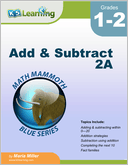Add and Subtract 2A Adding and subtracting within 0-20, adding doubles, subtraction strategies, completing the next ten, fact families and single digit subtraction facts.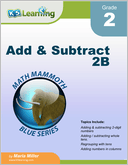Add and Subtract 2B Addition and subtraction within 0-100, both mentally and in columns, especially concentrating on regrouping ("carrying" in addition and "borrowing" in subtraction).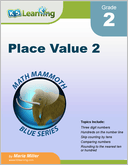Place Value 2 Three digit numbers; place values of ones, tens and hundreds.  Number lines, skip counting by tens, rounding to the nearest ten or hundred, bar graphs and picture graphs.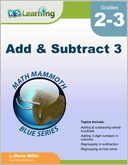Add & Subtract 3 Adding and subtracting 3-digit numbers, mentally and in columns, within 1-1,000. Includes regrouping tens as hundreds and regrouping across zeros. Also ordinal numbers, roman numerals and order of operations.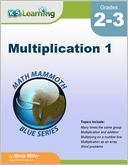Multiplication 1 Introducing the concept of multiplication and the order of operations.  The second part of the book focuses on the memorization of the multiplication tables.  Includes word problems.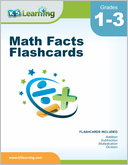Math Facts Flashcards Flashcards for addition, subtraction, multiplication and division facts, 0-12.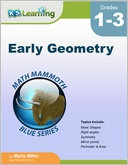Early Geometry Basic shapes (rectangles, squares,...), right angles, symmetry, mirror points, perimeter and area.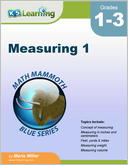Measuring 1 Length, weight and capacity for grades 1-3, including the concept of measuring lengths, weights and volumes.  Both customary and metric systems are used.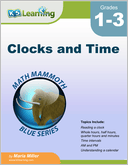Reading a clock, time intervals, am & pm and understanding a calendar.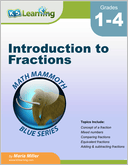Introduction to Fractions Introducing fractions, mostly for grades 3-4. Concept of a fraction, mixed numbers, comparing fractions, equivalent fractions, adding and subtracting fractions and fractions multiplied by a whole number.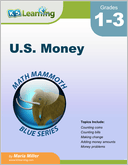U.S. Money Recognizing coins and bills; counting money, making change and money problems. We also have similar books in other currencies (Canadian money, Australian Money, British Money, European Money and South African Money).